Definition: mode, in statistics from The Columbia Encyclopedia

in statistics, an infrequently used type of average. In a group of numbers the mode is the number occurring most frequently. In the group 1, 4, 5, 5, 6, 6, 6, 6, 9, 9, the mode is 6 because it occurs four times and the others only once or twice.

Summary Article: Mode
From Encyclopedia of Research Design

Together with the mean and the median, the mode is one of the main measurements of the central tendency of a sample or a population. The mode is particularly important in social research because it is the only measure of central tendency that is relevant for any data set. That being said, it rarely receives a great deal of attention in statistics courses. The purpose of this entry is to identify the role of the mode in relation to the median and the mean for summarizing various types of data.

Definition

The mode is generally defined as the most frequent observation or element in the distribution. Unlike the mean and the median, there can be more than one mode. A sample or a population with one mode is unimodal. One with two modes is bimodal, one with three modes is trimodal, and so forth. In general, if there is more than one mode, one can say that a sample or a distribution is multimodal.

History

The mode is an unusual statistic among those defined in the field of statistics and probability. It is a counting term, and the concept of counting elements in categories dates back prior to human civilization. Recognizing the maximum of a category, be it the maximum number of predators, the maximum number of food sources, and so forth, is evolutionarily advantageous.

The mathematician Karl Pearson is often cited as the first person to use the concept of the mode in a statistical context. Pearson, however, also used a number of other descriptors for the concept, including the “maximum of theory” and the “ordinate of maximum frequency.”

Calculation

In order to calculate the mode of a distribution, it is helpful first to group the data into like categories and to determine the frequency of each observation. For small samples, it is often easy to find the mode by looking at the results. For example, if one were to roll a die 12 times and get the following results,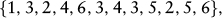it is fairly easy to see that the mode is 3.

However, if one were to roll the die 40 times and list the results, the mode is less obvious: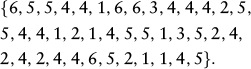In Table 1, the data are grouped by frequency, making it obvious that the mode is 4.

 Number Observations 1 6 2 6 3 2 4 13 5 9 6 4
 Classes (income by categories) Frequencies (number in each category) 0-\$20k 12 \$20k-\$40k 23 \$60k-\$60k 42 \$60k-\$80k 25 \$80k-\$100k 9 \$100k-\$120k 2 Total 113

Often, a statistician will be faced with a table of frequencies in which the individual data have been grouped into ranges of categories. Table 2 gives an example of such a table. We can see that the category of incomes between \$40,000 and \$60,000 has the largest number of members. We can call this the modal class. Yadolah Dodge has outlined a method for calculating a more precise estimate for the mode in such circumstances: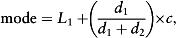where L1 = lower value of the modal category; d1 = difference between the number in the modal class and the class below; d2 = difference between the number in the modal class and the class above; and c = length of the interval within the modal class. (This interval length should be common for all intervals.)

In this particular example, the mode would be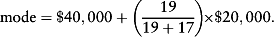Therefore, the mode would be estimated to be \$50,556.

The Mode for Various Types of Data Classification
Discrete and Continuous Data

In mathematical statistics, distributions of data are often discussed under the heading of discrete or continuous distributions. As the previous examples have shown, computing the mode is relatively straightforward with discrete data, although some organizing of the data may be necessary.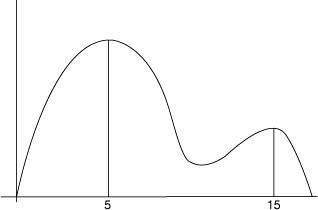Figure 1 Example of Modes for Continuous Distributions

For continuous data, the concept of mode is less obvious. No value occurs more than once in continuous probability distributions, and therefore, no single value can be defined as the mode with the discrete definition. Instead, for continuous distributions, the mode occurs at a local maximum in the data. For example, in Figure 1, the modes occur at 5 and 15.

As Figure 1 shows, for continuous distributions, a distribution can be defined as multimodal even when the local maximum at 5 is greater than the local maximum at 15.

The Stevens Classification System

In social science statistical courses, data are often classified according to data scales outlined by Stanley Smith Stevens.

According to Stevens's classification system, data can be identified as nominal, ordinal, interval, or ratio. As Stevens points out, the mode is the only measure of central tendency applicable to all data scales.

Data that comply with ratio scales can be either continuous or discrete and are amenable to all forms of statistical analysis. In mathematical terms, data can be drawn from the real, integer, or natural number systems. Although the mode applies as a measure of central tendency for such data, it is not usually the most useful measurement of central tendency. An example of ratio-scale data would be the total net worth of a randomly selected sample of individuals. So much variability is possible in the possible outcomes that unless the data are grouped into discrete categories (say increments of \$5,000 or \$10,000) the mode does not summarize the central tendency of the data well by itself.

Interval data are similar to ratio data in that it is possible to carry out detailed mathematical operations on them. As a result, it is possible to take the mean and the median as measures of central tendency. However, interval data lack a true 0 value.

For example, in measuring household size, it is conceivable that a household can possess very large numbers of members, but generally this is rare. Many households have fewer than 10 members; however, some modern extended families might run well into double digits. At the extreme, it is possible to observe medieval royal or aristocratic households with potentially hundreds of members. However, it is nonsensical to state that a household has zero members. It is also nonsensical to say that a specific household has 1.75 members. However, it is possible to say that the mean household size in a geographic region (a country, province, or city) is 2.75 or 2.2 or some other number. For interval data, the mode, the median, and the mean frequently provide valuable but different information about the central tendencies of the data. The mean may be heavily influenced by large low-prevalence values in the data; however, the median and the mode are much less influenced by them.

As an example of the role of the mode in summarizing interval data, if a researcher were interested in comparing the household size on different streets, A Street and B Street, he or she might visit both and record the following household sizes: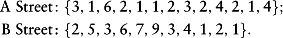Comparing the measures of central tendency (A Street: Mean = 2.5, Median = 2, Mode = 1 and 2; B Street: Mean = 3.8, Median = 3, Mode = 3) gives a clearer picture of the nature of the streets than any one measure of central tendency in isolation.

For data in ordinal scales, not only is there no absolute zero, but one can also rank the elements only in order of value. For example, a person could be asked to rank items on a scale of 1 to 5 in terms of his or her favorite. Likert-type scales are an example of this sort of data. A value of 5 is greater than a value of 4, but an increment from 4 to 5 does not necessarily represent the same increase in preference that an increase from 1 to 2 does. Because of these characteristics, reporting the median and the mode for this type of data makes sense, but the mean does not.

In the case of nominal-scale data, the mode is the only meaningful measure of central tendency. Nominal data tell the analyst nothing about the order of the data. In fact, data values do not even need to be labeled as numbers. One might be interested in which number a randomly selected group of hockey players at a hockey camp wear on their jersey back home in their regular hockey league. One could select from two groups: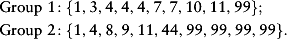For these groups, taking the mean and the median are meaningless as measures of central tendency (the number 11 does not represent more value than 4). However, the mode of Group 1 is 4, and the mode of Group 2 is 99. With some background information about hockey, the analyst could hypothesize which populations the two groups are drawn from. Group 2 appears to be made up of a younger group of players whose childhood hero is Wayne Gretzky (number 99), and Group 1 is likely made up of an older group of fans of Bobby Orr (number 4).

Another interesting property of the mode is that the data do not actually need to be organized as numbers, nor do they need to be translated into numbers. For example, an analyst might be interested in the first names of CEOs of large corporations in the 1950s. Examining a particular newspaper article, the analyst might find the following names: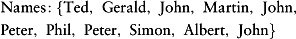In this example, the mode would be John, with three listings. As this example shows, the mode is particularly useful for textual analysis. Unlike the median and the mean, it is possible to take counts of the occurrence of words in documents, speech, or database files and to carry out an analysis from such a starting point.

Examination of the variation of nominal data is also possible by examining the frequencies of occurrences of entries. In the above example, it is possible to summarize the results by stating that John represents 3/11 (27.3%) of the entries, Peter represents 2/11 (18.2%) of the entries and that each other name represents 1/11 (9.1%) of the entries. Through a comparison of frequencies of occurrence, a better picture of the distribution of the entries emerges even if one does not have access to other measures of central tendency.

A Tool for Measuring the Skew of a Distribution

Skew in a distribution is a complex topic; however, comparing the mode to the median and the mean can be useful as a simple method of determining whether data in a distribution are skewed. A simple example is as follows. In a workplace a company offers free college tuition for the children of its employees, and the administrator of the plan is interested in the amount of tuition that the program may have to pay. For simplicity, take three different levels of tuition. Private university tuition is set at \$30,000, out-of-state-student public university tuition is set at \$15,000, and in-state tuition is set at \$5,000.

In this example, 10 students qualify for tuition coverage for the current year. The distribution of tuition amounts for each student is as follows: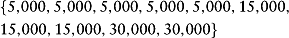Hence, the mode is \$5,000. The median is equal to (15,000 + 5,000)/2 = \$10,000. The mean= \$13,000. The tuition costs are skewed toward low tuition, and therefore the distribution has a negative skew.

A positively skewed distribution would occur if the tuition payments were as follows: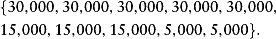Now the mode is \$30,000, the median is (30,000 + 15,000)/2 = \$22,500, and the mean = \$20,500.

Finally, the following observations are possible: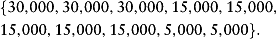In this case, the mode is \$15,000, the median is \$15,000, and the mean is \$17,500. The distribution has much less skew and is nearly symmetric.

The concept of skew can be dealt with using very complex methods in mathematical statistics; however, often simply reporting the mode together with the median and the mean is a useful method of forming first impressions about the nature of a distribution.

Central Tendency, Measures of, Interval Scale, Likert Scaling, Mean, Median, Nominal Scale

• (1993). Statistique: Dictionnaire encyclopédique [Statistics: Encyclopedic dictionary]. Paris: Dunod.
• Karl Pearson and the establishment of mathematical statistics. International Statistical Review 77 (1) : 3-29., 2009.
• Contribution to the mathematical theory of evolution: Skew variation in homogenous material. Philosophical Transactions of the Royal Society of London 186 (1) : 344-434., 1895.
• On the theory of scales of measurement. Science 103 (2684) : 677-680., 1946.

•Copyright © 2010 by SAGE Publications, Inc.

### Related Articles

##### Full text Article Mode
Encyclopedia of Measurement and Statistics

The mode is one characteristic of a distribution. It is the most frequently occurring value in a group of values. The mode can be an informative...

##### Full text Article mode
Dictionary of Economics, Wiley

The mode is determined by observation, usually in a graphic form. On such a graph, the modal value is the value of the point of highest...

##### Full text Article mode
The Blackwell Dictionary of Sociology

Like the mean and median , the mode is a statistical measure of the average score in a distribution . It is simply the most common score....

See more from Credo